SEARCH HOMEMath Central Quandaries & QueriesQuestion from Jolie, a student: Hello, I am trying to figure out what height from the ground a person's eyes are if they have a 12 degree visual angle to a screen that is 58cm away from their eyes? Thank you.Hi Jolie,

You currently do not have enough information to be able to calculate the eye level height. In the following diagram H is the height of the screen, E is the eye level height, D is the distance from the viewer to the screen, h is the visual angle and e is the angle of depression (angle from the line of sight to the bottom of the screen).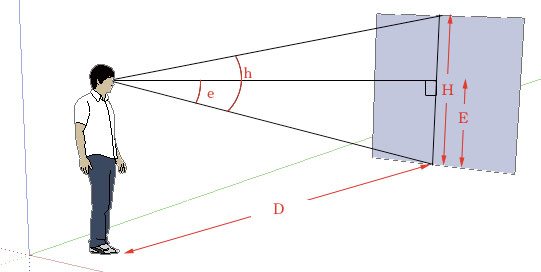The formula for visual angle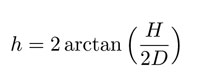can be rearranged to solve for the height of the screen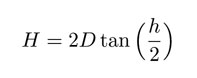We can use the following relation between the height of screen H and the eye level height E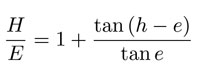then rearrange it to solve for E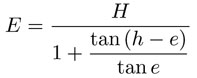As you can see, we are missing the angle of depression e so eye level height cannot be solved.

Hope this helps,

JaniceMath Central is supported by the University of Regina and The Pacific Institute for the Mathematical Sciences.